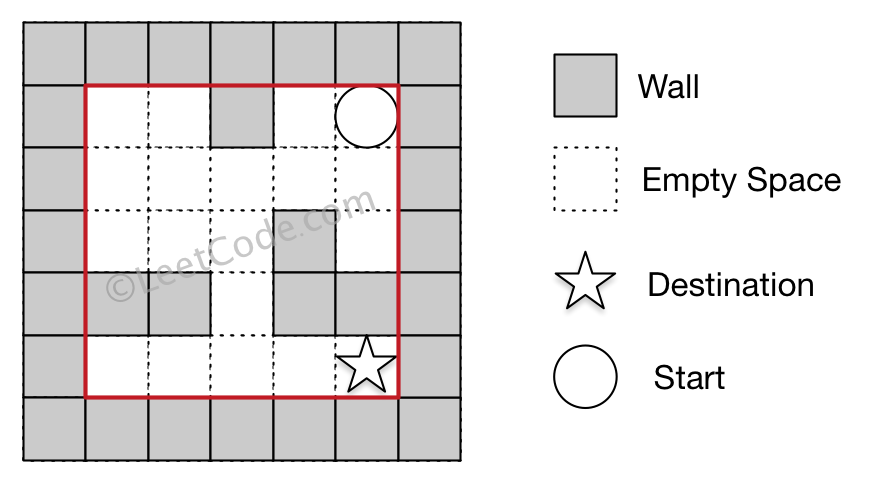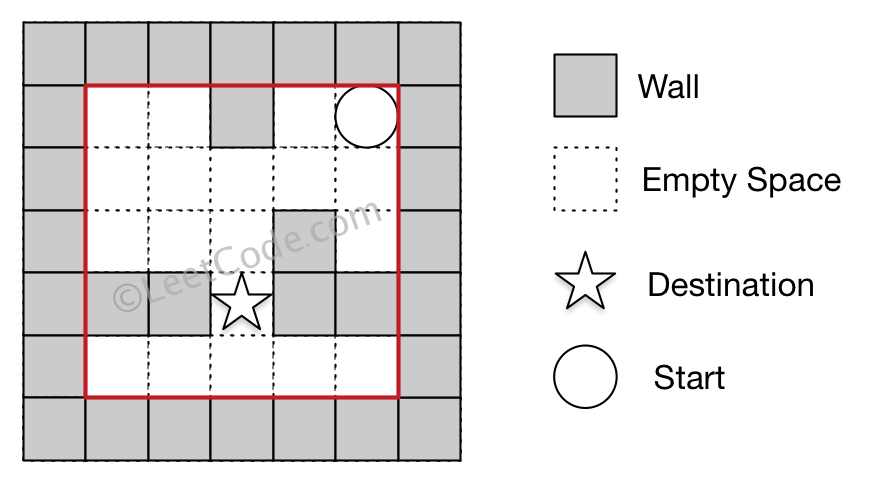Formatted question description: https://leetcode.ca/all/490.html

# 490. The Maze (Medium)

There is a ball in a maze with empty spaces and walls. The ball can go through empty spaces by rolling up, down, left or right, but it won't stop rolling until hitting a wall. When the ball stops, it could choose the next direction.

Given the ball's start position, the destination and the maze, determine whether the ball could stop at the destination.

The maze is represented by a binary 2D array. 1 means the wall and 0 means the empty space. You may assume that the borders of the maze are all walls. The start and destination coordinates are represented by row and column indexes.

Example 1:

Input 1: a maze represented by a 2D array

0 0 1 0 0
0 0 0 0 0
0 0 0 1 0
1 1 0 1 1
0 0 0 0 0

Input 2: start coordinate (rowStart, colStart) = (0, 4)
Input 3: destination coordinate (rowDest, colDest) = (4, 4)

Output: true

Explanation: One possible way is : left -> down -> left -> down -> right -> down -> right.Example 2:

Input 1: a maze represented by a 2D array

0 0 1 0 0
0 0 0 0 0
0 0 0 1 0
1 1 0 1 1
0 0 0 0 0

Input 2: start coordinate (rowStart, colStart) = (0, 4)
Input 3: destination coordinate (rowDest, colDest) = (3, 2)

Output: false

Explanation: There is no way for the ball to stop at the destination.Note:

1. There is only one ball and one destination in the maze.
2. Both the ball and the destination exist on an empty space, and they will not be at the same position initially.
3. The given maze does not contain border (like the red rectangle in the example pictures), but you could assume the border of the maze are all walls.
4. The maze contains at least 2 empty spaces, and both the width and height of the maze won't exceed 100.

Related Topics: Depth-first Search, Breadth-first Search

Similar Questions:

## Solution 1. BFS

// OJ: https://leetcode.com/problems/the-maze/
// Time: O(MN)
// Space: O(MN)
class Solution {
private:
int dirs = { {-1, 0}, {1, 0}, {0, -1}, {0, 1} };
int hash(vector<int> &p) { return p * 1000 + p; }
vector<int> go(vector<vector<int>>& maze, int x, int y, int d) {
int M = maze.size(), N = maze.size();
do {
x += d;
y += d;
} while (x >= 0 && x < M && y >= 0 && y < N && !maze[x][y]);
return { x - d, y - d };
}
public:
bool hasPath(vector<vector<int>>& maze, vector<int>& start, vector<int>& destination) {
queue<int> q;
unordered_set<int> seen;
int s = hash(start);
q.push(s);
seen.insert(s);
while (q.size()) {
int val = q.front(), x = val / 1000, y = val % 1000;
q.pop();
if (x == destination && y == destination) return true;
for (auto &dir : dirs) {
auto to = go(maze, x, y, dir);
int h = hash(to);
if (seen.find(h) != seen.end()) continue;
seen.insert(h);
q.push(h);
}
}
return false;
}
};


Java

• class Solution {
public boolean hasPath(int[][] maze, int[] start, int[] destination) {
final int BLOCK = -1;
final int WHITE = 0;
final int GRAY = 1;
final int BLACK = 2;
int rows = maze.length, columns = maze.length;
int[][] colors = new int[rows][columns];
for (int i = 0; i < rows; i++) {
for (int j = 0; j < columns; j++) {
if (maze[i][j] == 1)
colors[i][j] = BLOCK;
}
}
int[][] directions = { {-1, 0}, {1, 0}, {0, -1}, {0, 1} };
colors[start][start] = GRAY;
queue.offer(start);
while (!queue.isEmpty()) {
int[] position = queue.poll();
int row = position, column = position;
for (int[] direction : directions) {
int deltaRow = direction, deltaColumn = direction;
int stopRow = row, stopColumn = column;
int curRow = row + deltaRow, curColumn = column + deltaColumn;
while (curRow >= 0 && curRow < rows && curColumn >= 0 && curColumn < columns && colors[curRow][curColumn] != BLOCK) {
stopRow = curRow;
stopColumn = curColumn;
curRow += deltaRow;
curColumn += deltaColumn;
}
if (stopRow == destination && stopColumn == destination)
return true;
if (colors[stopRow][stopColumn] == WHITE) {
colors[stopRow][stopColumn] = GRAY;
queue.offer(new int[]{stopRow, stopColumn});
}
}
colors[row][column] = BLACK;
}
return false;
}
}

• // OJ: https://leetcode.com/problems/the-maze/
// Time: O(MN)
// Space: O(MN)
class Solution {
private:
int hash(vector<int> &p) { return p * 1000 + p; }
vector<int> go(vector<vector<int>>& maze, int x, int y, int d) {
int M = maze.size(), N = maze.size();
do {
x += d;
y += d;
} while (x >= 0 && x < M && y >= 0 && y < N && !maze[x][y]);
return { x - d, y - d };
}
public:
bool hasPath(vector<vector<int>>& maze, vector<int>& start, vector<int>& destination) {
queue<int> q;
unordered_set<int> seen;
int s = hash(start), dirs = { {-1, 0}, {1, 0}, {0, -1}, {0, 1} };
q.push(s);
seen.insert(s);
while (q.size()) {
int val = q.front(), x = val / 1000, y = val % 1000;
q.pop();
if (x == destination && y == destination) return true;
for (auto &dir : dirs) {
auto to = go(maze, x, y, dir);
int h = hash(to);
if (seen.count(h)) continue;
seen.insert(h);
q.push(h);
}
}
return false;
}
};

• from collections import deque

class Solution(object):
def hasPath(self, maze, start, destination):
"""
:type maze: List[List[int]]
:type start: List[int]
:type destination: List[int]
:rtype: bool
"""

def next(curr, maze):
height = len(maze)
width = len(maze)
directions = [(-1, 0), (1, 0), (0, -1), (0, 1)]
for di, dj in directions:
dist = 0
i, j = curr
while 0 <= i + di < height and 0 <= j + dj < width and maze[i + di][j + dj] != 1:
i += di
j += dj
dist += 1
yield (i, j)

queue = deque([tuple(start)])
visited = set()
destination = tuple(destination)
while queue:
curr = queue.popleft()
if curr in visited:
continue
if curr == destination:
return True
visited |= {curr}
for nbr in next(curr, maze):
queue.append(nbr)
return False